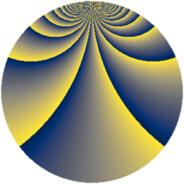# Properties

 Label 2300.2.bkLevel $2300$ Weight $2$ Character orbit 2300.bk Rep. character $\chi_{2300}(41,\cdot)$ Character field $\Q(\zeta_{55})$ Dimension $2400$ Sturm bound $720$

# Related objects

## Defining parameters

 Level: $$N$$ $$=$$ $$2300 = 2^{2} \cdot 5^{2} \cdot 23$$ Weight: $$k$$ $$=$$ $$2$$ Character orbit: $$[\chi]$$ $$=$$ 2300.bk (of order $$55$$ and degree $$40$$) Character conductor: $$\operatorname{cond}(\chi)$$ $$=$$ $$575$$ Character field: $$\Q(\zeta_{55})$$ Sturm bound: $$720$$

## Dimensions

The following table gives the dimensions of various subspaces of $$M_{2}(2300, [\chi])$$.

Total New Old
Modular forms 14640 2400 12240
Cusp forms 14160 2400 11760
Eisenstein series 480 0 480

## Trace form

 $$2400q - 4q^{3} + 6q^{5} - 4q^{7} + 56q^{9} + O(q^{10})$$ $$2400q - 4q^{3} + 6q^{5} - 4q^{7} + 56q^{9} + 10q^{11} - 8q^{13} - 23q^{15} + 6q^{17} + 16q^{19} + 8q^{21} - 60q^{23} + 64q^{25} + 26q^{27} + 12q^{29} - 12q^{31} + 30q^{33} + 16q^{35} - 84q^{37} - 24q^{39} - 100q^{43} - 14q^{45} + 124q^{47} - 260q^{49} - 32q^{51} + 20q^{53} - 2q^{55} - 56q^{57} - 18q^{59} + 8q^{61} - 22q^{63} + 79q^{65} + 66q^{67} - 129q^{69} + 78q^{71} - 20q^{73} - 212q^{75} + 52q^{77} + 16q^{79} + 150q^{81} + 86q^{83} + 44q^{85} - 124q^{87} + 4q^{89} - 12q^{91} - 36q^{93} + 187q^{95} + 72q^{97} + 62q^{99} + O(q^{100})$$

## Decomposition of $$S_{2}^{\mathrm{new}}(2300, [\chi])$$ into newform subspaces

The newforms in this space have not yet been added to the LMFDB.

## Decomposition of $$S_{2}^{\mathrm{old}}(2300, [\chi])$$ into lower level spaces

$$S_{2}^{\mathrm{old}}(2300, [\chi]) \cong$$ $$S_{2}^{\mathrm{new}}(575, [\chi])$$$$^{\oplus 3}$$$$\oplus$$$$S_{2}^{\mathrm{new}}(1150, [\chi])$$$$^{\oplus 2}$$